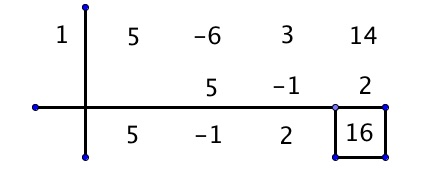# POLQ 1 | Lesson 2 | Explore (Synthetic Division)# Synthetic Division

***Synthetic division for polynomials only works when the divisor is of first degree and is in the form $$(x-a)$$. What that means is that if the divisor has a power greater than 1, this method won’t work!

Here are the steps for Synthetic Division as you saw in the video above:

1. Set the divisor equal to zero to find the number that will go in the left box. Make sure all of terms in the dividend are written in descending order.
2. Once the numbers are in the box, bring the leading coefficient of the dividend straight down.
3. Multiply the number in the left box by the number you brought down, and put the product in the next column under the second number. Add the two numbers together and put the sum below.
4. Repeat Step 3 until you have reached the end of the problem.
5. The final answer is determined by the bottom row. The numbers are the coefficients of the answer beginning with a power one less than the original dividend.

The example below is with no remainder. What would be different if there was a remainder other than zero?What if the divisor were $$x-1$$?Notice that there is a remainder of 16

The answer is $$5x^2-x+2+\dfrac{16}{x-1}$$